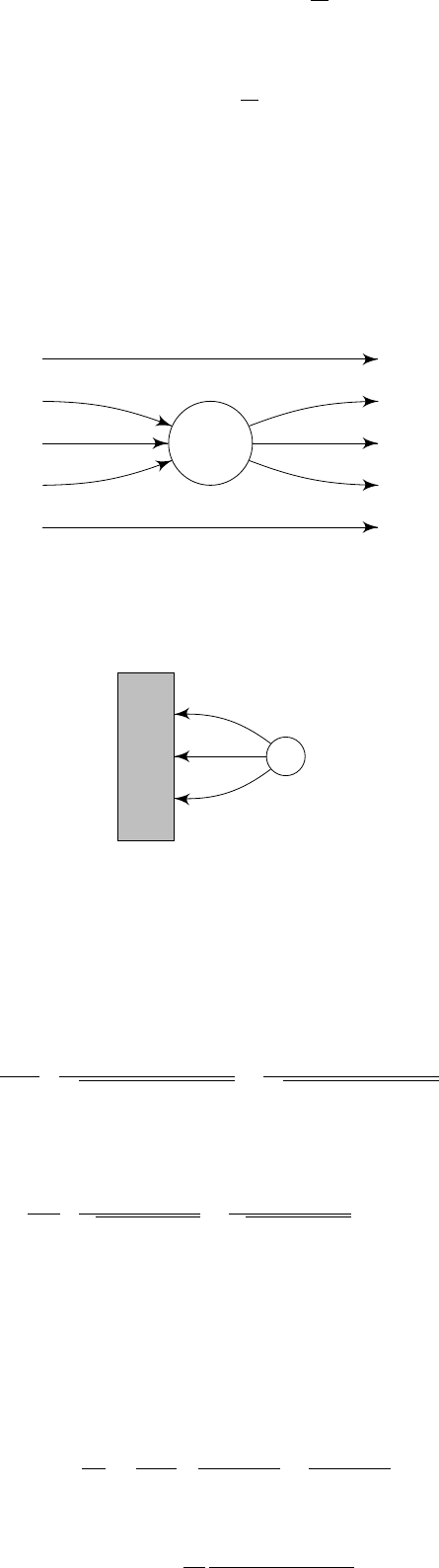2Electrostatics

IB Electromagnetism2.4 Conductors
Definition
(Conductor)
.
A conductor is a region of space which contains lots
of charges that are free to move.
In electrostatic situations, we must have
E
= 0 inside a conductor. Otherwise,
the charges inside the conductor would move till equilibrium. This almost
describes the whole of the section: if you apply an electric field onto a conductor,
the charges inside the conductor move until the external field is canceled out.
From this, we can derive a lot of results. Since
E
= 0,
φ
is constant inside
the conductor. Since
· E
=
ρ/ε
0
, inside the conductor, we must have
ρ
= 0.
Hence any net charge must live on the surface.
Also, since
φ
is constant inside the conductor, the surface of the conductor is
an equipotential. Hence the electric field is perpendicular to the surface. This
makes sense since any electric field with components parallel to the surface would
cause the charges to move.
Recall also from our previous discussion that across a surface, we have
ˆ
n · E
outside
ˆ
n · E
inside
=
σ
ε
0
,
where σ is the surface charge density. Since E
inside
= 0, we obtain
E
outside
=
σ
ε
0
ˆ
n
This allows us to compute the surface charge given the field, and vice versa.
Example.
Consider a spherical conductor with
Q
= 0. We put a positive plate
on the left and a negative plate on the right. This creates a field from the left to
the right.
With the conductor in place, since the electric field lines must be perpendicular
to the surface, they have to bend towards the conductor. Since field lines end
and start at charges, there must be negative charges at the left and positive
charges at the right.
+
We get an induced surface charge.
Example.
Suppose we have a conductor that fills all space
x <
0. We ground
it such that
φ
= 0 throughout the conductor. Then we place a charge
q
at
x = d > 0.
φ = 0 +
We are looking for a potential that corresponds to a source at
x
=
d
and satisfies
φ
= 0 for
x <
0. Since the solution to the Poisson equation is unique, we can
use the method of images to guess a solution and see if it works if it does, we
are done.
To guess a solution, we pretend that we don’t have a conductor. Instead, we
have a charge
q
and
x
=
d
. Then by symmetry we will get
φ
= 0 when
x
= 0.
The potential of this pair is
φ =
1
4πε
0
"
q
p
(x d)
2
+ y
2
+ z
2
q
p
(x + d)
2
+ y
2
+ z
2
#
.
To get the solution we want, we “steal” part of this potential and declare our
potential to be
φ =
1
4πε
0
q
(xd)
2
+y
2
+z
2
q
(x+d)
2
+y
2
+z
2
if x > 0
0 if x 0
Using this solution, we can immediately see that it satisfies Poisson’s equations
both outside and inside the conductor. To complete our solution, we need to find
the surface charge required such that the equations are satisfied on the surface
as well.
To do so, we can calculate the electric field near the surface, and use the
relation
σ
=
ε
0
E
outside
·
ˆ
n
. To find
σ
, we only need the component of
E
in the
x
direction:
E
x
=
φ
x
=
q
4πε
0
x d
|r d|
3/2
x + d
|r + d|
3/2
for x > 0. Then induced surface charge density is given by E
x
at x = 0:
σ = E
x
ε
0
=
q
2π
d
(d
2
+ y
2
+ z
2
)
3/2
.
The total surface charge is then given by
Z
σ dy dz = q.